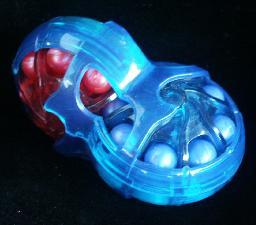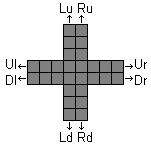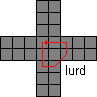# Rubik's ShellsRubik's Shells is a puzzle that consists of 4 intersecting rings, coloured wheels with 8 balls each, which can rotate. The wheels are in two pairs; two axes with a pair of wheels on each, and the two axes are separated and at right angles to each other so that any wheels from one axis has exactly one ball in common with any wheel from the other axis. The object of the puzzle is to separate the coloured balls, each colour into its own wheel.

There are 28 balls, 7 of each colour. There are 4 intersections, so 4 balls will belong to two wheels simultaneously. Each wheel intersects two others, so in the solved state each wheel will have one intersection of its own colour, and the ball at the other intersection matches that of the other wheel.

An interesting feature of this puzzle is that each axis also has a button. By pressing a button, the two wheels on that axis permanently lock together. There are therefore 3 grades of difficulty; Basic (no buttons pressed), Tough (one button pressed), and Challenge (both buttons pressed). Note that once pressed, the buttons can never be released (unless you drill a hole in the button and use a hook or screw to pull it back out). Also, the puzzle is always solvable, even if the buttons are pressed when it is in a mixed state. Pressing a button takes some force, so there is no danger of doing so accidentally.

## The number of positions:

There are 28 balls, which can be arranged in at most 28! ways. This limit is not reached because they fall into 4 groups of 7 indistinguishable balls. This means the number of positions is 28!/ 7!4 = 472,518,347,558,400. It should also be mentioned that there are actually two solutions, because the balls at the intersections can be arranged in two ways.

The booklet that comes with the puzzle has picture diagrams that show various puzzle positions. These diagrams show the balls in a cross, as if the rings were cut open. The two solved positions are shown in the following diagrams. I have added the letters b, g, w, r to indicate the 4 colours, so it remains legible when the page is printed.

 w b w b w b g g g w g g g g r r r r b r r r w b w b w b
 w b w b w b g g g g b g g g r r r w r r r r w b w b w b

If your browser supports JavaScript, then you can play Rubik's Shells by clicking the link below:

## JavaScript Rubik's Shells## Notation:

A notation for the moves will be useful, especially when describing the way to solve the last few balls on or near the intersections. Look at one of the diagrams above. The two vertical lines will be denoted by L and R, for the Left and Right column of balls. An up or down shift of one of these will then be denoted by Lu, Ld, Ru or Rd. Similarly, the up/down horizontal rows are U and D, and their moves are Ul, Ur, Dl and Dr. If two wheels are connected, then the letter indicating the wheel will be omitted.

## Solution, Basic game:

The Basic difficulty level (no buttons pressed) is very easy.

Phase 1: Solve the outer balls
In this phase everything but the 4 intersections will be solved.

1. Choose a wheel/colour that you want to solve. It is usually best to choose one which already has many balls of the right colour. I'll assume this it is wheel U - if it isn't, you can always reorient the puzzle so that it is.
2. Find a ball of the U colour, but which does not lie in the U wheel.
3. If the ball lies in wheel D, then turn D to bring it to an intersection. The ball can now be thought of as lying in wheel L or R instead. If one of L or R is already solved, then choose the intersection with the unsolved wheel.
4. Turn wheel U to bring one of its wrong balls to the intersection with the wheel that has the ball of colour U.
5. Turn the wheel containing the ball, bringing it into the U wheel.
6. Repeat steps b-e, until the U wheel is solved except for one or both of the intersections.
7. Repeat the above method for a wheel on the other axis.
8. Repeat the above method for each of the remaining wheels.

Phase 2: Solve the intersections.

1. Do any move that does not disturb any solved balls, and if possible, also solves one of the incorrect ones.
2. Step a can be repeated, choosing a wheel from a different axis each time, until the puzzle is solved.

At the start of phase 2 there are essentially only 4 different positions for the 4 balls at the intersections. Below is a list of diagrams of these positions with their solutions. Instead of colours, the letters A, B, C, D are used. The balls which are wrong (i.e. not in the wheel of the same colour) are shown in bold.

 C D A D A A B C B B C D
 C D A D A A B B C B C D
 C D A A B A B D C B C D
 C D A B C A B D A B C D
Ur Ur Dl Dr Rd Dl Dl
or Rd Dr Ru Dl
Ld Dl Lu Ul Dr

## Solution, Tough game:

The Tough difficulty level (one button pressed) is more of a real puzzle but still not too challenging. Two wheels are now fixed together, and can no longer move separately. I will assume that the fixed wheels are held so they are horizontal, and the free wheels are therefore vertical. In our notation, the D and U wheels are fixed together.

Phase 1: Solve the U wheel

1. Find a ball of U colour which does not lie in the U wheel.
2. If the ball is in the D wheel, then turn the UD wheels to bring it to an intersection, then shift the ball up into the U wheel. Continue with step e.
3. If the ball is in the L wheel, then:
1. If it is at the intersection of the L and D wheels, then first do Ld.
2. Turn wheels UD until the intersection of L and U is clear (has no U ball in it).
3. Turn wheel L until the ball is in the U wheel.
The ball has now been inserted in the U wheel, so continue with step e.
4. If the ball is in the R wheel, then do much the same as step c:
1. If it is at the intersection of R and D, then first do Rd.
2. Do wheels UD until the intersection of R and U is clear (has no U ball in it).
3. Turn wheel R until the ball is in the U wheel.
The ball has now been inserted in the U wheel, so continue with step e.
5. Repeat a-c until all the U balls are correctly placed in the U wheel. (Actually you only need to place 6 adjacent balls as you don't need to solve the intersections yet.)

Phase 2: Solve the D wheel

1. Find a ball of the D colour which is not in the U or D wheels.
2. If the ball lies in the R wheel, then:
1. Turn wheels UD until the intersection of R and U is empty (not a U ball).
2. Turn wheel R until the ball lies just below the D wheel.
3. Turn wheels UD until the intersection of R and D is empty (not a D ball), but the intersection of L and U does have a U ball. In other words, bring out a U ball that does not yet have a D ball below it.
4. Do moves l Ld r Ru.
The ball is now in position in the D wheel. Continue with step d.
3. If instead the ball lies in the L wheel, then:
1. Turn wheels UD until the intersection of L and U is empty (not a U ball).
2. Turn wheel L until the ball lies just below the D wheel.
3. Turn wheels UD until the intersection of L and D is empty (not a D ball), but the intersection of L and U does have a U ball. In other words, bring out a U ball that does not yet have a D ball below it.
4. Do moves r Rd l Lu.
The ball is now in position in the D wheel
4. Repeat a-c until all the D balls are correctly placed in the D wheel. (Actually you only need to place 6 adjacent balls as you don't need to solve the intersections yet.)
5. Turn the UD wheels so that their only unsolved balls are at the intersections.

Phase 3: Solve the free wheels.

1. Find a ball in the R wheel that does not belong there. Turn R to bring it to the intersection with the U wheel.
2. Find a ball in the L wheel that does not belong there. Turn L to bring it to the intersection with the U wheel.
3. Do moves Rd Rd r Ru l.
4. Repeat steps a-c until all the L and R balls are solved.
5. Turn the R and L wheels until the intersections are solved as well.

## Solution, Challenge game:

The Challenge difficulty level (two buttons pressed) is the most difficult. Both pairs of wheels are now fixed together, and can no longer move separately.This solution depends on the simple move sequence l u r d. These move leaves the ball at the intersection of D and R in position, but the seven balls adjacent to it are cycled anti-clockwise around it. By doing the inverse, u l d r, the same balls are cycled around clockwise. Of course, this sequence can also be performed in a different orientation to cycle the balls around one of the other intersections.

Phase 1: Solve 5 pairs of balls of one set of wheels
For now, only five adjacent pairs of balls on the UD wheels will be solved, because this still allows you some movement while solving the other set of wheels.

1. If you already have any pairs of U/D balls already solved, then turn the UD wheels to bring them just to the left of the intersections.
2. You will now put a ball of the D colour at the UL intersection as follows:
1. If there is a D ball somewhere in the L wheel, simply turn the LR wheels to bring it to the UL intersection.
2. If there is a D ball somewhere in the R wheel, simply turn the LR wheels to bring it to the UR intersection, and do moves l u r d and it will be at the UL intersection.
3. If there is a D ball in the U wheel, then do move l until it lies at the UL intersection, do move u, turn the UD wheels back to their original position, and do move d.
4. If there is a D ball in the D wheel (that is not yet part of a solved), then do move l until it lies at the DL intersection, do moves u u, turn the UD wheels back to their original position, and do move d.
3. Do move l.
4. Find a ball of the colour of the U wheel, and bring it to the UL intersection in the same way as step b above.
5. Do u r d l, to pair up the two balls.
6. Repeat steps b-e until there are 5 solved pairs.

Phase 2: Solve 5 pairs of balls of the other set of wheels
Note that to solve the LR wheels in this phase you will have to mess up the pair of balls immediately to the right of the intersections, so if by chance you actually have six solved pairs of balls (instead of 5) on the UD wheels, one pair will become mixed.
Remember that the move sequence l u r d cycles the 7 balls that lie around the DR intersection.

1. If you already have any pairs of L/R balls already solved, then turn the LR wheels to bring them just above the intersections.
2. You will now put a ball of the L colour at the UR intersection as follows:
1. If the ball is one of the 8 that lie adjacent to the DR intersection, then simply do repeat moves l u r d (or its inverse u l d r) until it lies at the UR intersection.
2. If the ball is not one of the 8 that lie adjacent to the DR intersection, then do u one or more times until it is. Then repeat the cycle l u r d until to bring the ball to a position to the right of the intersections. Now you can do d again until the LR wheels are back into their original position. The ball can be brought to the UR intersection by doing l u r d once or twice.
3. Do move u.
4. Find a ball of the colour of the R wheel, and bring it to the position immediately to the right of the UR intersection in the much same way as step b above.
5. Do d l u r, to pair up the two balls.
6. Repeat steps b-e until there are 5 solved pairs.

Phase 3: Solve the remaining balls next to the intersections.
The two balls immediately to the right and the two just below the intersections are solved in this phase.

1. Do l u r d as often as you like, to solve as many of the balls as is possible. It is nearly always possible to solve two of the four this way, and quite often three.
2. Choose one of the remaining unsolved positions adjacent to the intersections.
3. Rotate the whole puzzle so that the position you want to solve lies just below the DL intersection.
4. Find a ball at one of the intersections that is of the L colour (i.e. the colour that belongs at the position you are trying to solve). If there isn't such a ball, then go back to step b, choosing a different position to solve.
5. Put the ball into position by doing the appropriate move sequence below:
UL intersection: u l uu l dd r d l uuu r ddd r
UR intersection: l u l uu l dd r d l uuu r ddd rr
DL intersection: l u l uu r dd r d l uuu l ddd rr
DR intersection: ll u r d r u l u l d r u r d l d r
6. Repeat steps b-e until only the intersections remain to be solved.
 C D A C A B B X C D
 C D A C A B B X C D
 C D A A B C B X C D
 C D A A B C B X C D
uluulddrdluuurdddr luluulddrdluuurdddrr luluurddrdluuuldddrr llurdrululdrurdldr

Phase 4: Solve the balls at the intersections.

1. Count how many of the balls do not lie in the wheel of the same colour.
2. If only one ball is wrong, hold the puzzle so that this ball is at the UL intersection, and it belongs in the R wheel. (In the basic game, Ur would solve this position). Do moves u l dd l uu rr d l d r.
3. If two balls are wrong, hold the puzzle so that one of them lies at the UL intersection, and belongs in the R wheel. (In the basic game, Ur Dl would solve this position). Do moves l d rr d ll u rr u.
4. If three balls are wrong, hold the puzzle so that the correct one lies at the UL intersection, and is of the colour of the U wheel. Do moves rr d l u l d rr u l d.
5. If all four balls are wrong, hold the puzzle so that they need to be cycled around counter-clockwise. Do moves ll u rr uu ll d rr dd l.
 C D A D A A B C B B C D
 C D A D A A B B C B C D
 C D A A B A B D C B C D
 C D A B C A B D A B C D
ulddluurrdldr ldrrdllurru rrdluldrruld llurruulldrrddl